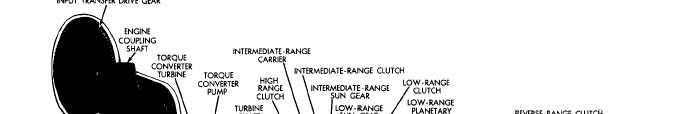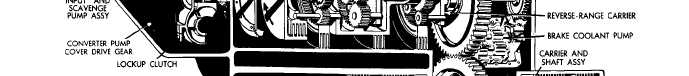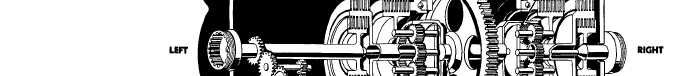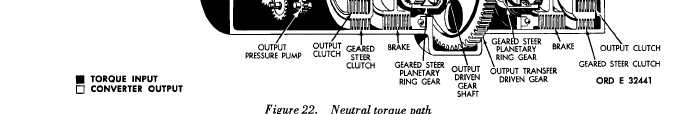Custom SearchFigure 22 P A R    4   6 T O R Q U E     P A T H S C H A P    2,   SEC  V 4 6 .    T O R Q U E    P A T H    -    N E U T R A L to   pars.   42   and   43,   above.   Since   clutches   are ( f i g .    2 2) not   applied,   no   torque   is   transmitted   beyond t h e    l o w -    a n d    i n t e r m e d i a t e - r a n g e    s u n    g e a r s. In   neutral,   torque   is   transmitted   to   the S o m e    o f    t h e    r a n g e    g e a r s    r o t a t e    f r e e l y ,    b u t, turbine   shaft   through   the   input   transfer   gears since   there   is   no   reaction,   no   torque   is   trans- and   torque   converter   (or   lockup   clutch).   Refer m i t t e d . 4 9Integrated Publishing, Inc. - A (SDVOSB) Service Disabled Veteran Owned Small Business